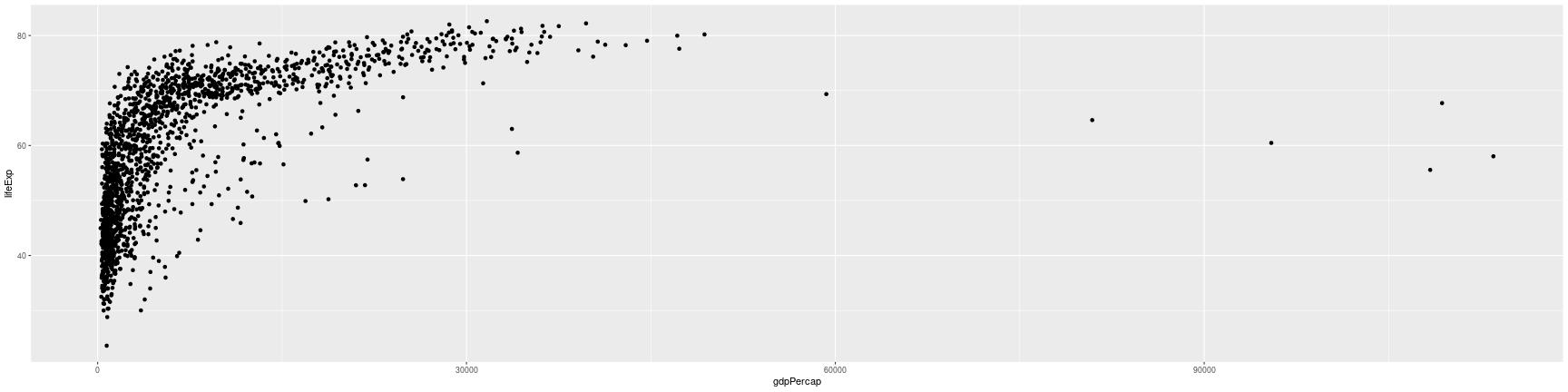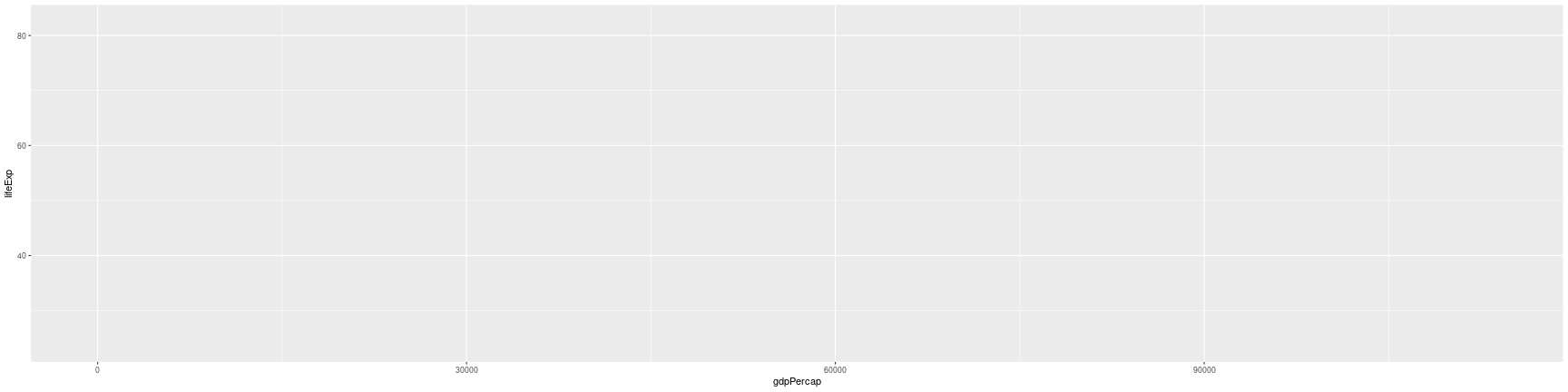## Instructions:

Use the left and right arrow keys to navigate the presentation forward and backward respectively. You can also use the arrows at the bottom right of the screen to navigate with a mouse.

FAIR USE ACT DISCLAIMER:
This site is for educational purposes only. This website may contain copyrighted material, the use of which has not been specifically authorized by the copyright holders. The material is made available on this website as a way to advance teaching, and copyright-protected materials are used to the extent necessary to make this class function in a distance learning environment. The Fair Use Copyright Disclaimer is under section 107 of the Copyright Act of 1976, allowance is made for “fair use” for purposes such as criticism, comment, news reporting, teaching, scholarship, education and research.

## Outline

• The following topics will be covered in this lecture:
• ggplot2 basics
• Graphics layers
• Transformations and statistics

• There are three main plotting systems in R,
1. the base plotting system which we have seen already;
2. the lattice package;
3. and the ggplot2 package.
• For the rest of the session, we’ll learn about the ggplot2 package, because it is the common plotting library in R for creating publication quality graphics.
• ggplot2 is built on the idea that any plot can be expressed from the same set of components:
1. a data set,
2. a coordinate system, and
3. a set of geoms – the visual representation of data points.
• The key to understanding ggplot2 is thinking about a figure in layers.
• This idea may be familiar to you if you have used image editing programs like Photoshop, Illustrator, or Inkscape.
• We will begin by loading the gapminder data again along with ggplot2:
require(gapminder)
require(ggplot2)


### ggplot2 basics

• Let's start off with an example:
ggplot(data = gapminder, mapping = aes(x = gdpPercap, y = lifeExp)) +
geom_point()• The first thing we do is call the ggplot function.

• This function lets R know that we're creating a new plot, and any of the arguments we give the ggplot function are the global options for the plot:

• i.e., they apply to all layers on the plot.

### ggplot2 basics

ggplot(data = gapminder, mapping = aes(x = gdpPercap, y = lifeExp)) +
geom_point()• We've passed in two arguments to ggplot.

• First, we tell ggplot what data we want to show on our figure, in this example the gapminder data we read in earlier.

• For the second argument, we passed in the aes function, which tells ggplot how variables in the data map to aesthetic properties of the figure;

• in this case the aesthetic properties are the x and y locations.
• Here we told ggplot we want to plot the “gdpPercap” column on the x-axis and the “lifeExp” column on the y-axis.

### ggplot2 basics

ggplot(data = gapminder, mapping = aes(x = gdpPercap, y = lifeExp)) +
geom_point()• Notice that we didn't need to explicitly pass aes these columns (e.g. x = gapminder[, "gdpPercap"]);

• this is because ggplot will look in the dataframe for that column.

### ggplot2 basics

• By itself, the call to ggplot isn't enough to draw a figure:
ggplot(data = gapminder, mapping = aes(x = gdpPercap, y = lifeExp))• We need to tell ggplot how we want to visually represent the data, which we do by adding a new geom layer.

• In our example, we used geom_point, which tells ggplot we want to visually represent the relationship between x and y as a scatterplot of points.

### Layers

• Using a scatterplot probably isn't the best for visualizing change over time.

• Instead, let's tell ggplot to visualize the data as a line plot:

ggplot(data = gapminder, mapping = aes(x=year, y=lifeExp, by=country, color=continent)) +
geom_line()